JMSLTM Numerical Library 7.2.0
com.imsl.math

Class CsShape

• All Implemented Interfaces:
Serializable, Cloneable

public class CsShape
extends Spline
Extension of the Spline class to interpolate data points consistent with the concavity of the data.

Class CsShape computes a cubic spline interpolant to n data points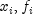for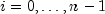. For ease of explanation, we will assume that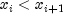, although it is not necessary for the user to sort these data values. If the data are strictly convex, then the computed spline is convex,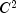, and minimizes the expressionover all convex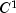functions that interpolate the data. In the general case when the data have both convex and concave regions, the convexity of the spline is consistent with the data and the above integral is minimized under the appropriate constraints. For more information on this interpolation scheme, we refer the reader to Micchelli et al. (1985) and Irvine et al. (1986).

One important feature of the splines produced by this class is that it is not possible, a priori, to predict the number of breakpoints of the resulting interpolant. In most cases, there will be breakpoints at places other than data locations. The method is nonlinear; and although the interpolant is a piecewise cubic, cubic polynomials are not reproduced. (However, linear polynomials are reproduced.) This routine should be used when it is important to preserve the convex and concave regions implied by the data.